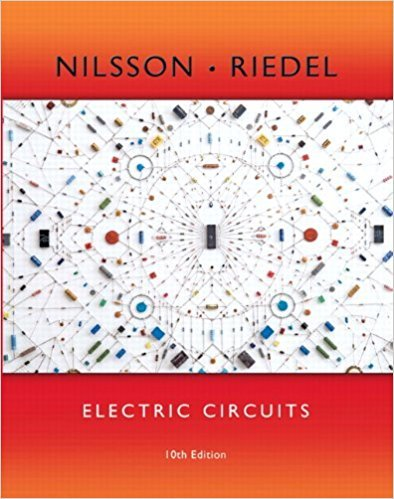×
×

# Solutions for Chapter 3.5: Measuring Voltage and Current## Full solutions for Electric Circuits | 10th Edition

ISBN: 9780133760033Solutions for Chapter 3.5: Measuring Voltage and Current

Solutions for Chapter 3.5
4 5 0 344 Reviews
30
4
##### ISBN: 9780133760033

This expansive textbook survival guide covers the following chapters and their solutions. Since 2 problems in chapter 3.5: Measuring Voltage and Current have been answered, more than 13092 students have viewed full step-by-step solutions from this chapter. Chapter 3.5: Measuring Voltage and Current includes 2 full step-by-step solutions. Electric Circuits was written by and is associated to the ISBN: 9780133760033. This textbook survival guide was created for the textbook: Electric Circuits, edition: 10.

Key Engineering and Tech Terms and definitions covered in this textbook
×